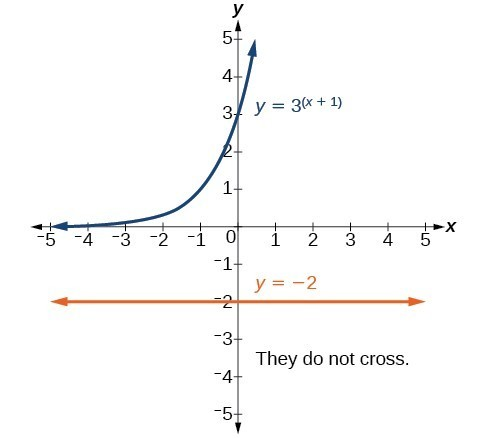## Use like bases to solve exponential equations

The first technique involves two functions with like bases. Recall that the one-to-one property of exponential functions tells us that, for any real numbers b, S, and T, where $b>0,\text{ }b\ne 1$, ${b}^{S}={b}^{T}$ if and only if = T.

In other words, when an exponential equation has the same base on each side, the exponents must be equal. This also applies when the exponents are algebraic expressions. Therefore, we can solve many exponential equations by using the rules of exponents to rewrite each side as a power with the same base. Then, we use the fact that exponential functions are one-to-one to set the exponents equal to one another, and solve for the unknown.

For example, consider the equation ${3}^{4x - 7}=\frac{{3}^{2x}}{3}$. To solve for x, we use the division property of exponents to rewrite the right side so that both sides have the common base, 3. Then we apply the one-to-one property of exponents by setting the exponents equal to one another and solving for x:

$\begin{cases}{3}^{4x - 7}\hfill & =\frac{{3}^{2x}}{3}\hfill & \hfill \\ {3}^{4x - 7}\hfill & =\frac{{3}^{2x}}{{3}^{1}}\hfill & {\text{Rewrite 3 as 3}}^{1}.\hfill \\ {3}^{4x - 7}\hfill & ={3}^{2x - 1}\hfill & \text{Use the division property of exponents}\text{.}\hfill \\ 4x - 7\hfill & =2x - 1\text{ }\hfill & \text{Apply the one-to-one property of exponents}\text{.}\hfill \\ 2x\hfill & =6\hfill & \text{Subtract 2}x\text{ and add 7 to both sides}\text{.}\hfill \\ x\hfill & =3\hfill & \text{Divide by 3}\text{.}\hfill \end{cases}$

### A General Note: Using the One-to-One Property of Exponential Functions to Solve Exponential Equations

For any algebraic expressions S and T, and any positive real number $b\ne 1$,

${b}^{S}={b}^{T}\text{ if and only if }S=T$

### How To: Given an exponential equation with the form ${b}^{S}={b}^{T}$, where S and T are algebraic expressions with an unknown, solve for the unknown.

1. Use the rules of exponents to simplify, if necessary, so that the resulting equation has the form ${b}^{S}={b}^{T}$.
2. Use the one-to-one property to set the exponents equal.
3. Solve the resulting equation, = T, for the unknown.

### Example 1: Solving an Exponential Equation with a Common Base

Solve ${2}^{x - 1}={2}^{2x - 4}$.

### Solution

$\begin{cases} {2}^{x - 1}={2}^{2x - 4}\hfill & \text{The common base is }2.\hfill \\ \text{ }x - 1=2x - 4\hfill & \text{By the one-to-one property the exponents must be equal}.\hfill \\ \text{ }x=3\hfill & \text{Solve for }x.\hfill \end{cases}$

### Try It 1

Solve ${5}^{2x}={5}^{3x+2}$.

Solution

## Rewriting Equations So All Powers Have the Same Base

Sometimes the common base for an exponential equation is not explicitly shown. In these cases, we simply rewrite the terms in the equation as powers with a common base, and solve using the one-to-one property.

For example, consider the equation $256={4}^{x - 5}$. We can rewrite both sides of this equation as a power of 2. Then we apply the rules of exponents, along with the one-to-one property, to solve for x:

$\begin{cases}256={4}^{x - 5}\hfill & \hfill \\ {2}^{8}={\left({2}^{2}\right)}^{x - 5}\hfill & \text{Rewrite each side as a power with base 2}.\hfill \\ {2}^{8}={2}^{2x - 10}\hfill & \text{Use the one-to-one property of exponents}.\hfill \\ 8=2x - 10\hfill & \text{Apply the one-to-one property of exponents}.\hfill \\ 18=2x\hfill & \text{Add 10 to both sides}.\hfill \\ x=9\hfill & \text{Divide by 2}.\hfill \end{cases}$

### How To: Given an exponential equation with unlike bases, use the one-to-one property to solve it.

1. Rewrite each side in the equation as a power with a common base.
2. Use the rules of exponents to simplify, if necessary, so that the resulting equation has the form ${b}^{S}={b}^{T}$.
3. Use the one-to-one property to set the exponents equal.
4. Solve the resulting equation, = T, for the unknown.

### Example 2: Solving Equations by Rewriting Them to Have a Common Base

Solve ${8}^{x+2}={16}^{x+1}$.

### Solution

$\begin{cases}\text{ }{8}^{x+2}={16}^{x+1}\hfill & \hfill \\ {\left({2}^{3}\right)}^{x+2}={\left({2}^{4}\right)}^{x+1}\hfill & \text{Write }8\text{ and }16\text{ as powers of }2.\hfill \\ \text{ }{2}^{3x+6}={2}^{4x+4}\hfill & \text{To take a power of a power, multiply exponents}.\hfill \\ \text{ }3x+6=4x+4\hfill & \text{Use the one-to-one property to set the exponents equal}.\hfill \\ \text{ }x=2\hfill & \text{Solve for }x.\hfill \end{cases}$

### Try It 2

Solve ${5}^{2x}={25}^{3x+2}$.

Solution

### Example 3: Solving Equations by Rewriting Roots with Fractional Exponents to Have a Common Base

Solve ${2}^{5x}=\sqrt{2}$.

### Solution

$\begin{cases}{2}^{5x}={2}^{\frac{1}{2}}\hfill & \text{Write the square root of 2 as a power of }2.\hfill \\ 5x=\frac{1}{2}\hfill & \text{Use the one-to-one property}.\hfill \\ x=\frac{1}{10}\hfill & \text{Solve for }x.\hfill \end{cases}$

### Try It 3

Solve ${5}^{x}=\sqrt{5}$.

Solution

### Q & A

Do all exponential equations have a solution? If not, how can we tell if there is a solution during the problem-solving process?

No. Recall that the range of an exponential function is always positive. While solving the equation, we may obtain an expression that is undefined.

### Example 4: Solving an Equation with Positive and Negative Powers

Solve ${3}^{x+1}=-2$.

### Solution

This equation has no solution. There is no real value of x that will make the equation a true statement because any power of a positive number is positive.

### Analysis of the Solution

The figure below shows that the two graphs do not cross so the left side is never equal to the right side. Thus the equation has no solution.Figure 1

### Try It 4

Solve ${2}^{x}=-100$.

Solution